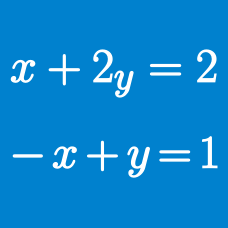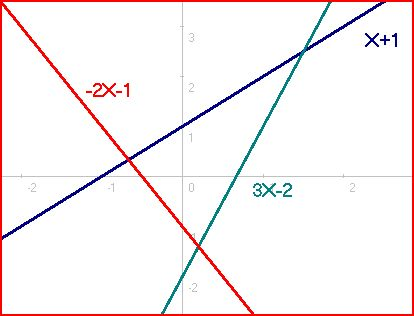Algebra

# System of Equations: Level 5 Challenges

$\begin{cases} 4\dfrac{\sqrt{x^{2} + 1}}{x} = 5\dfrac{\sqrt{y^{2} + 1}}{y}=6\dfrac{\sqrt{z^{2} + 1}}{z} \\ xyz = x + y + z\end{cases}$

If $x = \dfrac{\sqrt{a}}{b} , y = c\dfrac{\sqrt{d}}{e} , z = f\sqrt{g}$ satisfy the above system of equations, then find $(a + b + \ldots + g )$.

• The expression is in simplest terms, meaning that all of the variables are positive integers, $a, d, g$ are square free and $c, e$ are coprime.

Let $a$ and $b$ be positive integers such that $a\ge\ b$ and$\frac{a+1}{b}+\frac{b+1}{a}$is also a positive integer. Find sum of all possible values of $a$ which are less than 1000.The system of equations $4a^4+36a^2b^2+9b^4=20a^2+30b^2-1$ $2a^3b+3ab^3=5ab$ has $n$ nonnegative (meaning both $a$ and $b$ are nonnegative) real solutions. Let these solutions be $(a_1,b_1),(a_2,b_2),\cdots (a_n,b_n)$. The value of the sum $\sum_{i=1}^n a_i+b_i$ can be expressed in the form $\dfrac{a+b\sqrt{c}}{d}$ where $\gcd (a,b,d)=1$ and $c$ is squarefree. Find $a+b+c+d$.

A positive real number is given. In each move, we can either add $3$ to it, subtract $3$ to it, multiply it by $3$ and divide it by $3$. The sum of all numbers such that after exactly $3$ moves, the original number comes back is $\frac{a}{b}$, where $a$ and $b$ are relatively prime positive integers. Find $a + b$.

Find the largest possible value of $x^3+y^3+z^3$ for real $x,\ y,\ z,$ such that $\begin{cases} xyz^2=-64y-128x\\ x^2yz=-32y-32z\\ 3xy^2z=128x-64z \end{cases}$

×